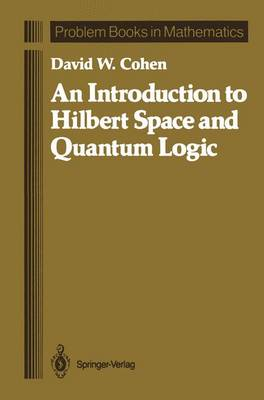•# An Introduction to Hilbert Space and Quantum Logic - Problem Books in Mathematics (Paperback)

(author)
£64.99
Paperback 149 Pages / Published: 12/10/2011
• We can order this from the publisher

Usually dispatched within 15 working days

Historically, nonclassical physics developed in three stages. First came a collection of ad hoc assumptions and then a cookbook of equations known as "quantum mechanics". The equations and their philosophical underpinnings were then collected into a model based on the mathematics of Hilbert space. From the Hilbert space model came the abstaction of "quantum logics". This book explores all three stages, but not in historical order. Instead, in an effort to illustrate how physics and abstract mathematics influence each other we hop back and forth between a purely mathematical development of Hilbert space, and a physically motivated definition of a logic, partially linking the two throughout, and then bringing them together at the deepest level in the last two chapters. This book should be accessible to undergraduate and beginning graduate students in both mathematics and physics. The only strict prerequisites are calculus and linear algebra, but the level of mathematical sophistication assumes at least one or two intermediate courses, for example in mathematical analysis or advanced calculus. No background in physics is assumed.

Publisher: Springer-Verlag New York Inc.
ISBN: 9781461388432
Number of pages: 149
Weight: 261 g
Dimensions: 235 x 155 x 9 mm
Edition: Softcover reprint of the original 1st ed. 198